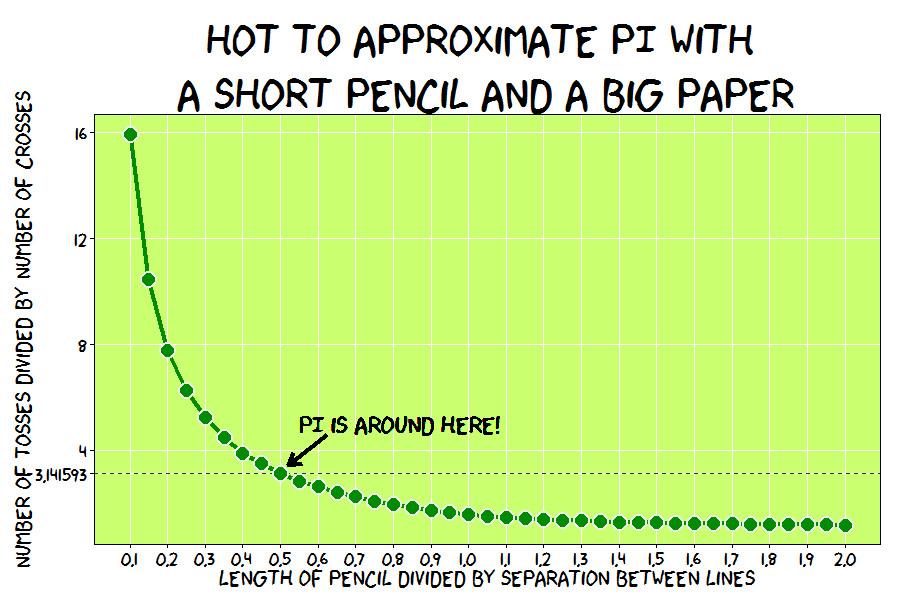# How To Approximate Pi With A Short Pencil And A Big Paper

Experiment, be curious: though interfering friends may frown, get furious at each attempt to hold you down (Tony Bennett, Experiment)

Instructions:

1. Take a pencil and measure it
2. Take a piece of paper and draw parallel lines on it (you can use the pencil, of course); separation between lines should double the length of the pencil
3. Toss the pencil over the paper 100 times (or more)
4. Make note of how many times do the pencil cross some of the lines
5. Calculate ratio between tosses and crosses: this is your approximation of Pi

Some time ago, I published a post about one of the most amazing places where PI was discovered. This is another example of the ubiquity of this mathematical constant. This experiment is based on Buffon’s needle problem, another amazing experiment of 18th century. Next plot represents ratio of tosses to crosses depending on the length of pencil. When the pencil is half the length of the separation between lines, the previous ratio is approximately PI:If you get very bored some afternoon you can replicate this experiment with your children. Use a short pencil. If not, you will need an extremely big piece of paper. Meanwhile here you have the code:

```trials=100000
results=sapply(seq(.1, 2, by = .05), function(x)
{
r=x #Length of pencil in relation to separation between lines
Needles=t(sapply(1:trials, function(y) c(100*runif(1),2*pi*runif(1))))
Needles=cbind(Needles,Needles[,1]+r*cos(Needles[,2]))
Needles=data.frame(x1=Needles[,1], x2=Needles[,3], Cross=abs(trunc(Needles[,1])-trunc(Needles[,3])))
c(r, trials/(trials-nrow(Needles[Needles\$Cross==0,])))
})
results=data.frame(t(results))
colnames(results)=c(c("ratio", "inv.perc.crosses"))
require(ggplot2)
require(extrafont)
require(grid)
opts=theme(
panel.background = element_rect(fill="darkolivegreen1"),
panel.border = element_rect(colour="black", fill=NA),
axis.line = element_line(size = 0.5, colour = "black"),
axis.ticks = element_line(colour="black"),
panel.grid.major = element_line(colour="white", linetype = 1),
panel.grid.minor = element_blank(),
axis.text.y = element_text(colour="black", size=20),
axis.text.x = element_text(colour="black", size=20),
text = element_text(size=25, family="xkcd"),
legend.key = element_blank(),
legend.position = c(.2,.75),
legend.background = element_blank(),
plot.title = element_text(size = 50))
c=ggplot(results, aes(ratio, inv.perc.crosses))
c +geom_abline(intercept = pi, slope = 0, size = 0.4, linetype=2, colour = "black", alpha=0.8)+
geom_line(color="green4", size=1.5)+
geom_point(color="gray92", size=8, pch=16)+
geom_point(color="green4", size=6, pch=16)+
geom_text(aes(0.55, 5), hjust=0, family="xkcd", label="PI is around here!", size=10)+
ggtitle("Hot to approximate PI with \na short pencil and a big paper")+
xlab("Length of pencil divided by separation between lines") +
ylab("Number of tosses divided by number of crosses")+
geom_segment(aes(x = .625, y = 4.6, xend = .52, yend = 3.45), size=1.5, arrow = arrow(length = unit(0.5, "cm")))+
scale_y_continuous(breaks=c(pi, 4, 8, 12, 16), labels=c("3.141593","4","8","12","16"))+
scale_x_continuous(breaks=seq(.1, 2, by = .1))+opts
```